Inequalities I

When solving inequalities, keep the following facts in mind:if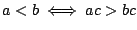iforEx 1 Solve the inequality.

Sol Subtracting 1 from each term gives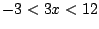, and then dividing by 3 gives. Therefore the solution is given by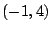.

Ex 2 Solve the inequality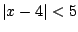.

Sol Writing the inequality without absolute values gives, and adding 4 to every term gives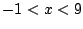. Thereforeis the solution.

Pr A Solve the inequality.

Pr B Solve the inequality.

Pr C Solve the double inequality.

Pr D Solve the double inequality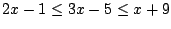.

Pr 1 Solve the inequality.

Pr 2 Solve the inequality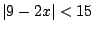.

Pr 3 Solve the inequality.

Pr 4 Solve the inequality.

Pr 5 Solve the inequality.

Pr 6 Solve the inequality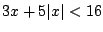.

Pr 7 Solve the inequality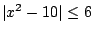.

Lawrence Marx 2002-07-12